﻿

The natural transformations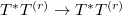$T^* T^{(r)}\to T^* T^{(r)}$

Abstract

For natural numbers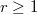$r\geq 1$ and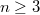$n\geq 3$ a complete classification of natural transformations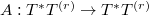$A:T^*T^{(r)}\to T^*T^{(r)}$ over$n$-manifolds is given, where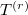$T^{(r)}$ is the linear$r$-tangent bundle functor.

DOI Code: 10.1285/i15900932v20n1p81

Keywords: Bundle functors; Natural transformations

Classification: 58A20; 53A55

Full Text: PDF